Cannon ball

We consider the problem of firing cannon balls: this involves two-dimensional motion in a gravitational field including air resistance. We set up Newton's equations for the x and y components of the motion.

g is the gravitational acceleration, and b the air drag coefficient. m is the mass of the ball. We assume the drag to be proportional to the velocity.

We set up Newton's equations as first-order differential equations for the momentum and position vector components (Hamilton's equation). In the x direction we have drag as the only force, while in the y direction gravity acts as well.

> restart;

> Momx:=m*diff(vx(t),t)=-b*vx(t);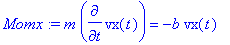> Momy:=m*diff(vy(t),t)=-m*g-b*vy(t);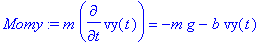The initial conditions are specified through the parameters:

> IC:=vx(0)=v0*cos(theta),vy(0)=v0*sin(theta);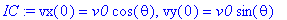> sol:=dsolve({Momx,Momy,IC},{vx(t),vy(t)});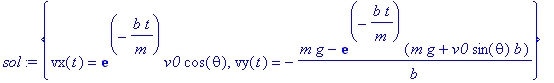> assign(sol);

We can verify that the found solutions indeed satisfy the differential equations:

> simplify(Momx);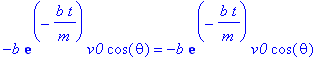> evalb(%);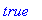> simplify(Momy);> evalb(%);It is of interest to Taylor expand the solutions around t =0. This shows how the velocities begin to deviate from their inital values:

> taylor(vx(t),t=0,3);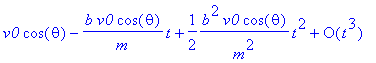> taylor(vy(t),t=0,3);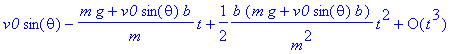The change is linear in time, and depends in the drag part on the initial velocity components, while the contributions from gravity are obvious in the linear term. Note that the quadratic terms are more complicated.

It is of interest to investigate various aspects of the solution. The most common problem concerns the dependence of the trajectory on the inclination of the cannon for fixed drag coefficient b . Note that in real life one will include additional factors, such as the wind speed and direction.

In order to plot solutions we need to specify the parameters. In SI (MKSA) units we have

> g:=9.81;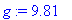we pick a mass of 1 kg:

> m:=1;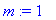a drag coefficient (in kg/s):

> b:=0.5;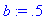> vx(t);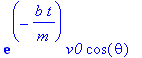> simplify(%);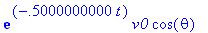> simplify(vy(t));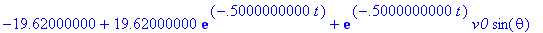Suppose we fix now the initial speed (by fixing the amount of powder for the firing of the cannon). We can still vary the inclination angle. How can we achieve this goal?

Again in SI units (in m/s):

> v0:=50;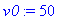We can choose a parameter for the inclination angle:

> simplify(vy(t));To generate functions rather than expressions we need the unapply command:

> Vx:=unapply(simplify(vx(t)),theta,t);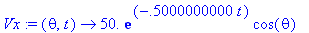> Vy:=unapply(simplify(vy(t)),theta,t);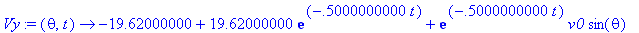We are ready to integrate the equations for the position vector components. A dummy variable has to be used for the time integration ( s replacing t ). Again we use the unapply procedure to generate a mapping.

> X:=unapply(int(Vx(theta,s),s=0..t),theta,t);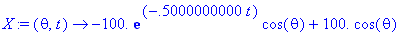> X(0.5,1);> Y:=unapply(int(Vy(theta,s),s=0..t),theta,t);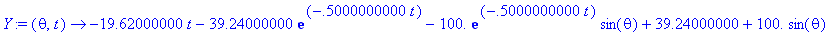> Y(0.5,1);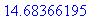We have functions that generate the position vector for given inclination angle theta (in radians) and time t (in seconds). Thus, we can plot the trajectory parametrically (the help page ?plot includes an example for this plot mode):

> plot([X(0.5,t),Y(0.5,t),t=0..10],x=0..X(0.5,10),y=0..20);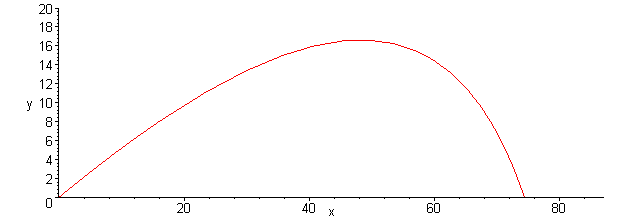The limits on the axes were adjusted by hand. If we wish to automate the process we can do this by finding the times at which the ball intercepts the surface.

This is done below.

As an exercise the reader may want to play around with the amount of friction. For a very small friction constant b the parabolic shape known from textbook solutions should be recovered.

The intercept with the surface is obtained from

> t0:=solve(Y(0.5,t)=0,t);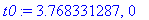Note that we cross y =0 at the beginning of the motion as well. The order in which the two solutions are found may vary. It does not matter for the parametric plot which way the trajectory is traced out. We do not need to specify the range on the axes, as they are automatically adjusted with the solution.

> plot([X(0.5,t),Y(0.5,t),t=t0..t0]);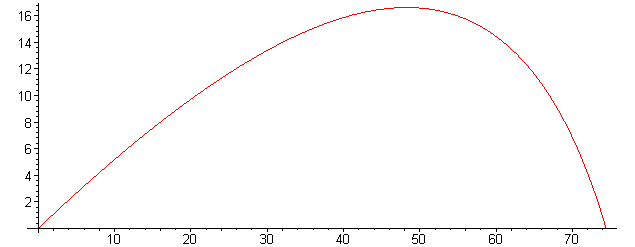We graph a family of solutions in which the inclination of the cannon is changed. The objective is to determine the range, and to observe the maximum height reached by the projectile.

> with(plots):

> imax:=5;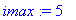We set up a color table to be able to distinguish the solutions.

> coltab:=[maroon,red,magenta,yellow,green,blue,violet,brown,gray,black];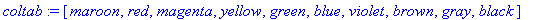> for i from 1 to imax do:

> theta:=(i-0.5)/imax*Pi/2;

> t0:=solve(Y(theta,t),t);

> P[i]:=plot([X(theta,t),Y(theta,t),t=t0..t0],color=coltab[i]): od:

> display(seq(P[i],i=1..imax));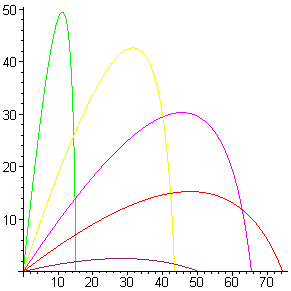We can also display the sequence of graphs as an animation:

> display(seq(P[i],i=1..imax),insequence=true);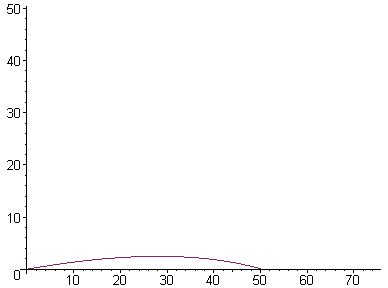An interesting extension is to vary the air drag as a function of the height. The air density falls off exponentially with increased height. The numerical solution of the generalized equation of motion which include a height-dependent air drag coefficient are discussed in N. Giordano's book Computational Physics (Prentice-Hall 1997).

>